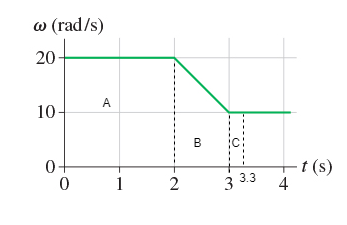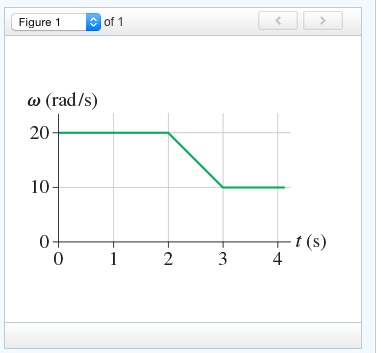# Problem: (Figure 1) shows the angular-velocity-versus-time graph for a particle moving in a circle. How many revolutions does the object make during the first 3.3 s?

###### FREE Expert Solution

The total angular distance is the area under the angular-velocity-versus-time graph.

Partition the graph into three parts as shown:85% (69 ratings)###### Problem Details

(Figure 1) shows the angular-velocity-versus-time graph for a particle moving in a circle. How many revolutions does the object make during the first 3.3 s?